# Stabilizer

(diff) ← Older revision | Latest revision (diff) | Newer revision → (diff)
of an elementin a setThe subgroup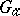of a group of transformations, operating on a set, consisting of the transformations that leave the elementfixed: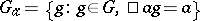. The stabilizer ofis also called the isotropy group of, the isotropy subgroup ofor the stationary subgroup of. If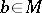,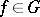and, then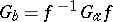. If one considers the action of the groupon itself by conjugation, the stabilizer of the elementwill be the centralizer of this element in; if the group acts by conjugation on the set of its subgroups, then the stabilizer of a subgroupwill be the normalizer of this subgroup (cf. Normalizer of a subset).
In caseis a set of mathematical structures, for instance a set of lattices in, on which a groupacts, for instance the group of Euclidean motions, then the isotropy subgroup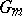of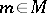is the symmetry group of the structure.